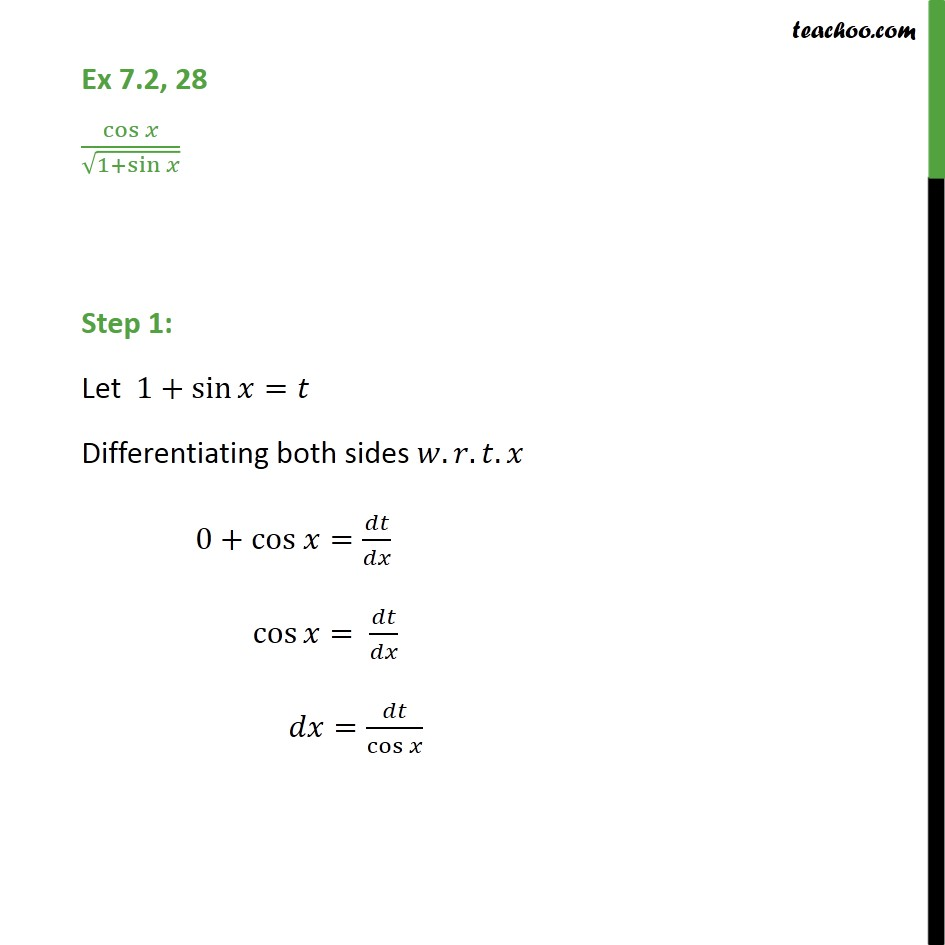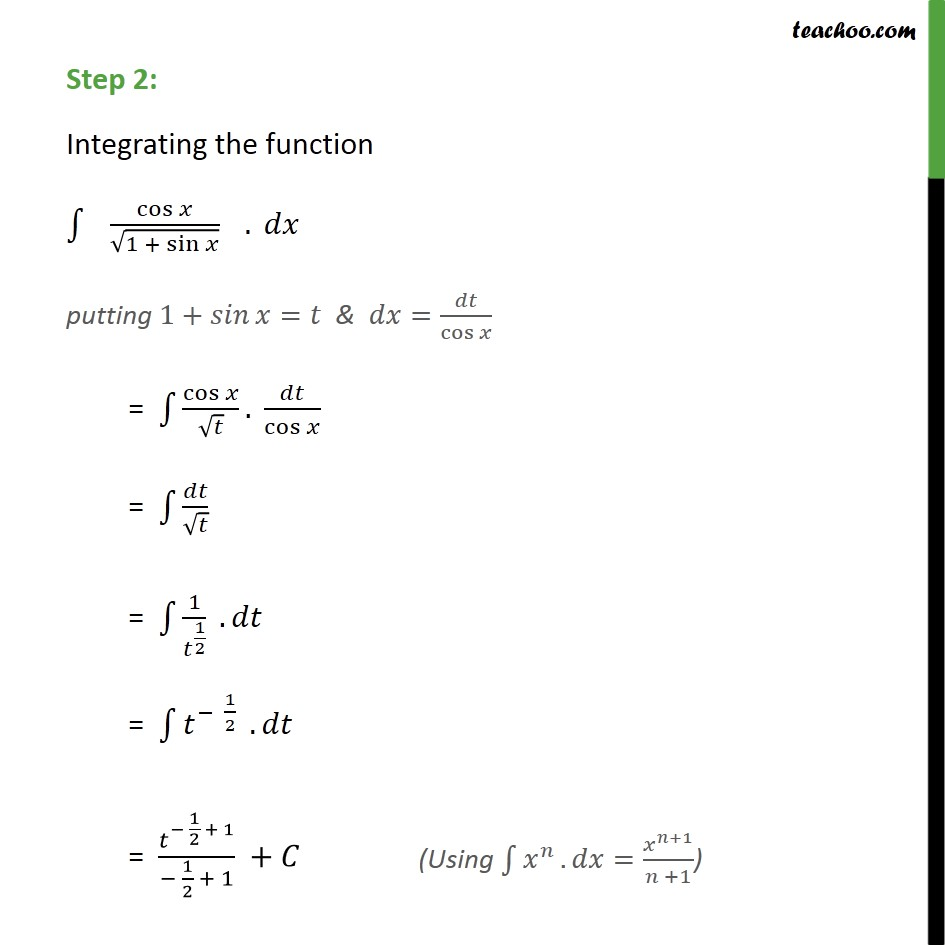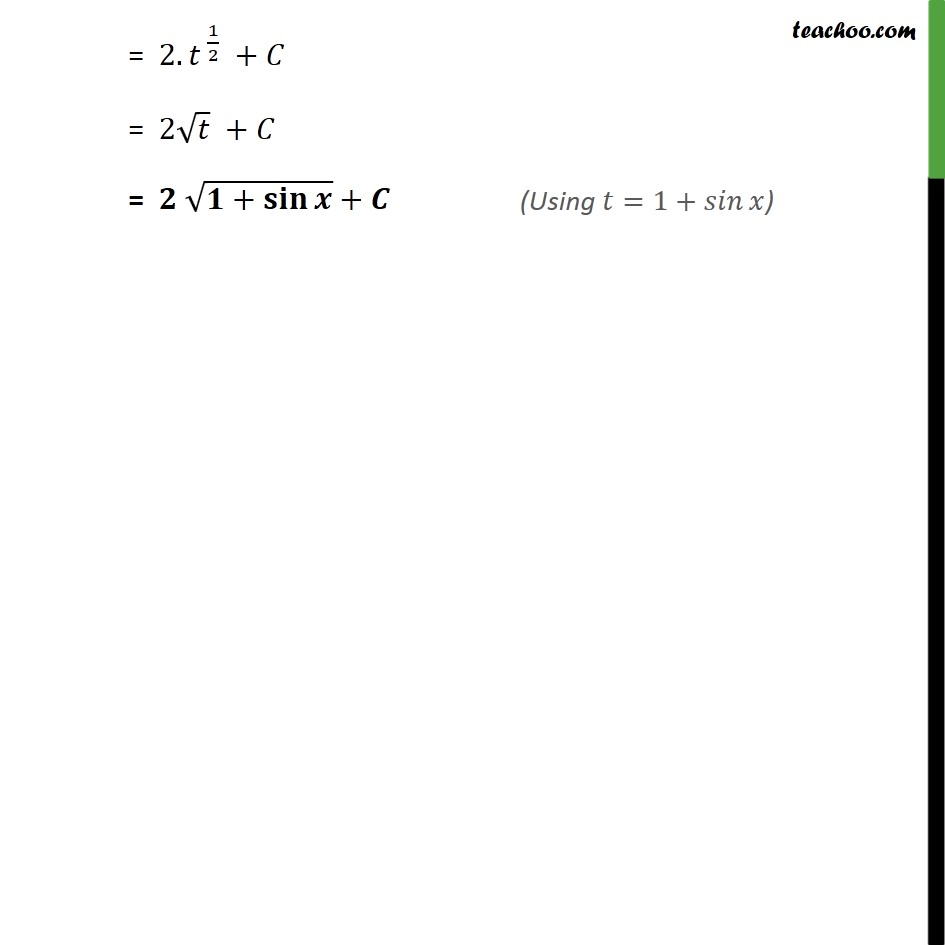1. Chapter 7 Class 12 Integrals
2. Serial order wise
3. Ex 7.2

Transcript

Ex 7.2, 28 cos﷮𝑥﷯﷮ ﷮1+ sin﷮𝑥﷯﷯﷯ Step 1: Let 1+ sin﷮𝑥﷯=𝑡 Differentiating both sides 𝑤.𝑟.𝑡.𝑥 0+cos 𝑥= 𝑑𝑡﷮𝑑𝑥﷯ cos﷮𝑥﷯= 𝑑𝑡﷮𝑑𝑥﷯ 𝑑𝑥= 𝑑𝑡﷮ cos﷮𝑥﷯﷯ Step 2: Integrating the function ﷮﷮ cos﷮𝑥﷯﷮ ﷮1 + sin﷮𝑥﷯﷯﷯ ﷯. 𝑑𝑥 putting 1+ 𝑠𝑖𝑛﷮𝑥﷯=𝑡 & 𝑑𝑥= 𝑑𝑡﷮ cos﷮𝑥﷯﷯ = ﷮﷮ cos﷮𝑥﷯﷮ ﷮𝑡﷯﷯﷯. 𝑑𝑡﷮ cos﷮𝑥﷯﷯ = ﷮﷮ 𝑑𝑡﷮ ﷮𝑡﷯﷯﷯ = ﷮﷮ 1﷮ 𝑡﷮ 1﷮2﷯﷯﷯ . 𝑑𝑡﷯ = ﷮﷮ 𝑡﷮− 1﷮2﷯ ﷯ . 𝑑𝑡﷯ = 𝑡﷮− 1﷮2﷯ + 1﷯﷮− 1﷮2﷯ + 1﷯ +𝐶 = 2. 𝑡﷮ 1﷮2﷯﷯ +𝐶 = 2 ﷮𝑡﷯ +𝐶 = 𝟐 ﷮𝟏+ 𝐬𝐢𝐧﷮𝒙﷯﷯+𝑪

Ex 7.2

Chapter 7 Class 12 Integrals
Serial order wise